## What fraction of 5 MeV α particles will be scattered through angles greater than 4.5° from a gold foil (Z = 79, density = 19.3 g/cm3) of thi

Question

What fraction of 5 MeV α particles will be scattered through angles greater than 4.5° from a gold foil (Z = 79, density = 19.3 g/cm3) of thickness 10-8 m?

in progress 0
5 months 2021-08-10T23:46:26+00:00 1 Answers 7 views 0

The fraction of particles is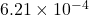Explanation:

Given :

Alpha particle atomic no.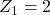Gold foil atomic number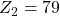Thickness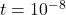m

Density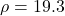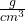Kinetic energy of alpha particle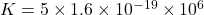V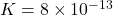V

Scattered angle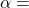4.5°

Fraction of incident particle scattered at angle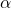is,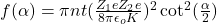Where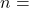number density of particle,For calculating value of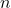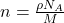Where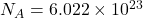,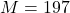( Mass number of gold foil )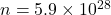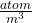Put the all value in above equation,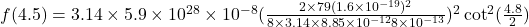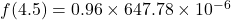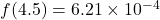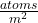Therefore, the fraction of particles is